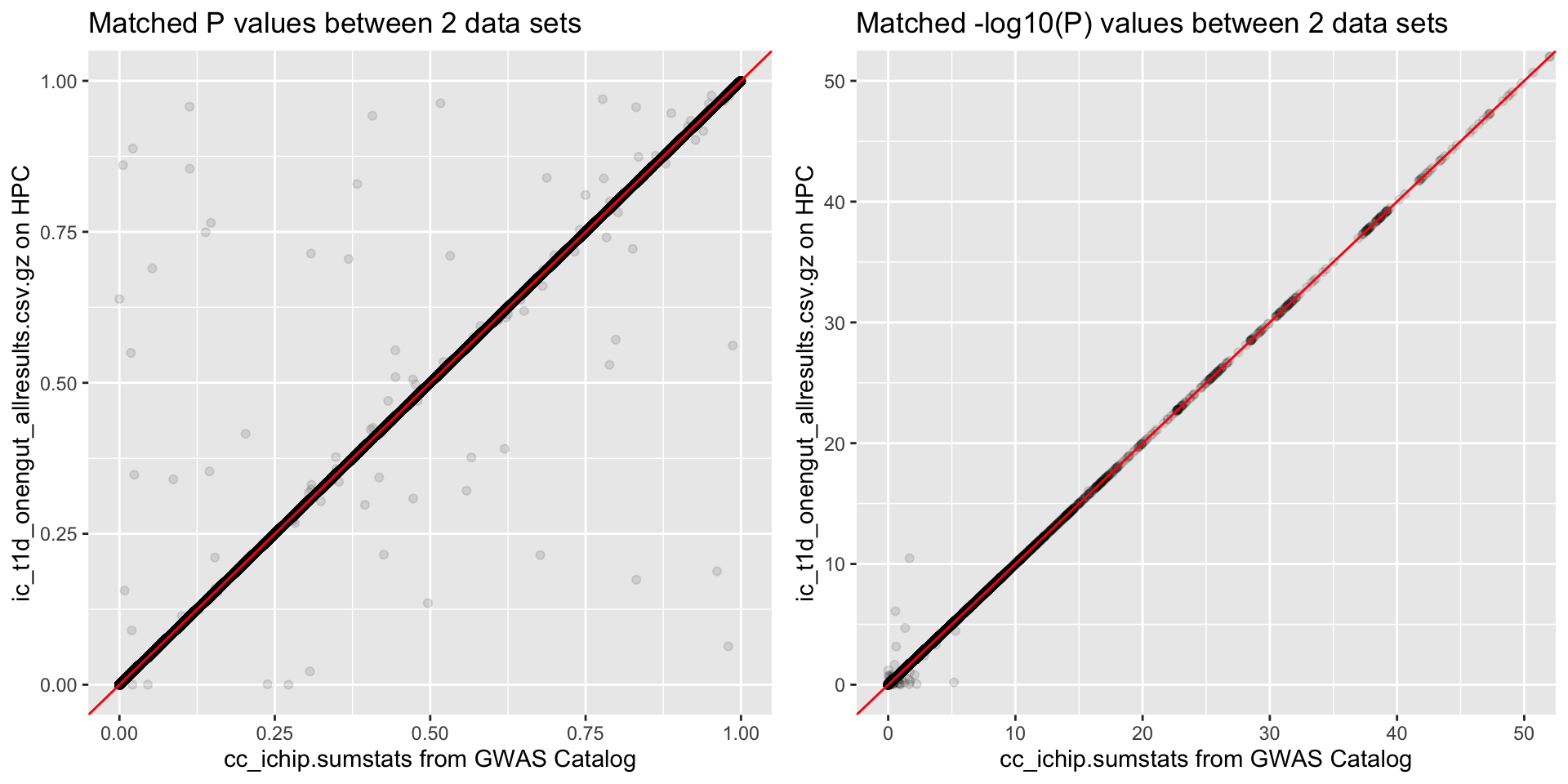## cFDR method for reweighting GWAS P values using functional data

### 1. Notation

$$H$$ denotes a hypothesis of association with T1D; $$H=1$$ indicates association, $$H=0$$ indicates no association (null hypothesis).

$\begin{equation} H \sim \text{Bernoulli}(\pi_1) \Longrightarrow P(H=1)=\pi_1 \end{equation}$

$\begin{equation} \begin{split} Q|H=0 \sim \text{Bernoulli}(q_0) & \Longrightarrow P(Q=1|H=0)=q_0 \\ & \Longrightarrow P(Q=0|H=0)=1-q_0 \end{split} \end{equation}$

$\begin{equation} P|H=0 \sim U(0,1) \end{equation}$

$\begin{equation} P \perp Q|H = 0 \end{equation}$

$\begin{equation} \begin{split} Q|H=1 \sim \text{Bernoulli}(q_1) & \Longrightarrow P(Q=1|H=1)=q_1 \\ & \Longrightarrow P(Q=0|H=1)=1-q_1 \end{split} \end{equation}$

$\begin{equation} f(P=p, Q=q|H_0)=f_0(p,q) \end{equation}$

$\begin{equation} f(P=p, Q=q|H_1)=f_1(p,q) \end{equation}$

$\begin{equation} f(P=p, Q=q)=f_0(p,q)(1-\pi_1)+f_1(p,q)\pi_1=f(p,q) \end{equation}$

### 2. Method

1. Generate the set of possible rejection regions.

Since $$Q$$ can only be 0 or 1, we just need two P value thresholds, $$p_0$$ for $$Q=0$$ and $$p_1$$ for $$Q=1$$.

$\begin{equation} L(p_0, p_1)=(P\leq p_0, Q=0) \cup (P\leq p_1, Q=1). \end{equation}$

2. Pick the smallest rejection region that $$(p[i], q[i])$$ belongs.

Following on from our aim, we want the rejection region such that:

1. the probability of a null instance of $$P,Q$$ falling inside $$L(p_0, p_1)$$ is fixed at some value $$\alpha$$, that is

$\begin{equation} \int_{L(p_0, p_1)}df_0=\alpha \end{equation}$

1. the probability of a non-null instance of $$P,Q$$ falling inside $$L(p_0, p_1)$$ is maximal, that is

$\begin{equation} \int_{L(p_0, p_1)}df_1 \text{ is maximal} \end{equation}$

Previous works (e.g. appendix 8.1 in James’ paper) show that this optimal region is given by the set of points $$\{(p,q):f_0(p,q)/f_1(p,q)\leq k_{\alpha}\}$$ which means that in our case, the chosen $$p_0$$ and $$p_1$$ should satisfy

$\begin{equation} \dfrac{f_0(p_0,0)}{f(p_0,0)}=\dfrac{f_0(p_1,1)}{f(p_1,1)} \end{equation}$

using $$f(p,q)=f_0(p,q)(1-\pi_1)+f_1(p,q)\pi_1$$.

To find $$p_0$$ and $$p_1$$ that satisfy this equation, we derive an estimator of

$\begin{equation} \begin{split} \dfrac{f_0(p,q)}{f(p,q)} &= \dfrac{f(P=p, Q=q|H_0)}{f(P=p, Q=q)}. \end{split} \end{equation}$

Let

$\begin{equation} \begin{split} \hat{h}(p,q) &= \dfrac{Pr(P\leq p, Q=q|H_0)}{Pr(P\leq p, Q=q)} \\[2ex] & = \dfrac{Pr(P\leq p| Q=q, H_0)Pr(Q=q|H_0)}{Pr(P\leq p|Q=q)Pr(Q=q)} \\[2ex] & \approx \dfrac{p\times Pr(Q=q|H_0)}{Pr(P\leq p|Q=q)Pr(Q=q)} \\[2ex] & \approx \dfrac{p\times \color{red}{A(q)}}{\color{blue}{B(p, q)}/N} \end{split} \end{equation}$

where $$\color{red}{A(q)=\dfrac{\# \{Q=q, p>1/2\}}{\# \{p>1/2\}}}$$ and $$\color{blue}{B(p, q)=\# \{P\leq p, Q = q\}}$$ (since $$\# \{Q = q\}$$ cancels in the denominator).

So we want to solve

$\begin{equation} \hat{h}(p_0,q = 0)=\hat{h}(p_1, q = 1) \end{equation}$

for

• $$p_1$$ if $$q=0$$ (set $$p_0=p_i$$)
• $$p_0$$ if $$q=1$$ (set $$p_1=p_i$$)

3. Integrate the null distribution over this region to get $$v$$.

$\begin{equation} \begin{split} \int_{L(p_0,p_1)}df_0 & = Pr(P,Q \in L(p_0, p_1)|H_0) \\ & = Pr((P\leq p_0, Q=0) \cup (P\leq p_1, Q=1) | H_0) \\ & = Pr(P\leq p_0, Q=0|H_0) + Pr(P\leq p_1, Q=1|H_1) \\ & = Pr(P\leq p_0|Q=0, H_0)Pr(Q=0|H_0) + Pr(P\leq p_1|Q=1, H_0)Pr(Q=1|H_0) \\ & = p_0\times (1-q_0) + p_1\times q_0 \end{split} \end{equation}$

where $$q_0=A(1)$$.

### 3. New data set

I have downloaded the case-control T1D GWAS results (Onengut-Gumuscu et al.) from the GWAS catalog. This consists of 123130 SNPs genotyped in 6670 T1D cases and 12262 controls.

I breifly compare this to the ic_t1d_onengut_allresults.csv.gz file on the HPC which I assume also includes the meta-analysis (124726 SNPs). For the SNPs that can be matched by position, I compare the reported P values in the two datasets.The T1D paper (cc_ichip.sumstats from GWAS Catalog) does not apply genomic control as we discussed (comparing chi-squared distributions) but does account for cyptic relatedness using KING (to estimate the kinship coefficient and remove problematic individuals) and population structure using the PCA method in KING. I assume this means that I shouldn’t genome-control the P values (again). Note: I checked, and this data set gives a lambda value of 0.93 suggesting that there is no population structure (although shouldn’t this equal 1 in a completely homogenous population?).

I decide to use the publically avaliable data downloaded directly from the GWAS catalog for my analysis.

### 4. Annotation data set

To derive a final annotation data set, for each of the 123130 SNPs in the data set, I record their reported genomic annotation in 19 different cell types (mostly immune related except brain cells to use as a control) using the segway encyclopedia. For each cell type, I also constuct a binary “active” column that is 1 if the SNP falls in enhancer, transcribed, promoter or reg permissive, 0 if the SNP falls in quiescent, constit het or facult het and NA if the SNP falls in bivalent, unclassified or low confidence.

snps <- readRDS("final_final_annots.RDS")
snps[1,]
##   chrom     pos       rsid other_allele effect_allele         p
## 1     1 1118275 rs61733845            C             T 0.8342689
##          beta         se     OR  OR_lower OR_upper
## 1 -0.01126319 0.05383169 0.9888 0.8897869 1.098831
##   BRAIN_INFERIOR_TEMPORAL_LOBE CD14_PRIMARY_CELLS
## 1               FacultativeHet     FacultativeHet
##   CD19_PRIMARY_CELLS_PERIPHERAL_UW CD3_PRIMARY_CELLS_CORD_BI
## 1                         Bivalent                  Bivalent
##   CD3_PRIMARY_CELLS_PERIPHERAL_UW CD4_MEMORY_PRIMARY_CELLS
## 1                  FacultativeHet                 Enhancer
##   CD4_NAIVE_PRIMARY_CELLS CD4+_CD25-_CD45RA+_NAIVE_PRIMARY_CELLS
## 1           RegPermissive                               Enhancer
##   CD4+_CD25-_CD45RO+_MEMORY_PRIMARY_CELLS
## 1                                Enhancer
##   CD4+_CD25-_IL17-_PMA-IONOMYCIN_STIMULATED_MACS_PURIFIED_TH_PRIMARY_CELLS
## 1                                                           FacultativeHet
##   CD4+_CD25-_IL17+_PMA-IONOMCYIN_STIMULATED_TH17_PRIMARY_CELLS
## 1                                                     Enhancer
##   CD4+_CD25-_TH_PRIMARY_CELLS CD4+_CD25+_CD127-_TREG_PRIMARY_CELLS
## 1                    Bivalent                       FacultativeHet
##   CD4+_CD25INT_CD127+_TMEM_PRIMARY_CELLS CD56_PRIMARY_CELLS
## 1                               Enhancer           Bivalent
##   CD8_MEMORY_PRIMARY_CELLS CD8_NAIVE_PRIMARY_CELLS   PANISLETS    THYMUS
## 1           FacultativeHet           RegPermissive Transcribed Quiescent
##   b_BRAIN_INFERIOR_TEMPORAL_LOBE b_CD14_PRIMARY_CELLS
## 1                              0                    0
##   b_CD19_PRIMARY_CELLS_PERIPHERAL_UW b_CD3_PRIMARY_CELLS_CORD_BI
## 1                                 NA                          NA
##   b_CD3_PRIMARY_CELLS_PERIPHERAL_UW b_CD4_MEMORY_PRIMARY_CELLS
## 1                                 0                          1
##   b_CD4_NAIVE_PRIMARY_CELLS b_CD4+_CD25-_CD45RA+_NAIVE_PRIMARY_CELLS
## 1                         1                                        1
##   b_CD4+_CD25-_CD45RO+_MEMORY_PRIMARY_CELLS
## 1                                         1
##   b_CD4+_CD25-_IL17-_PMA-IONOMYCIN_STIMULATED_MACS_PURIFIED_TH_PRIMARY_CELLS
## 1                                                                          0
##   b_CD4+_CD25-_IL17+_PMA-IONOMCYIN_STIMULATED_TH17_PRIMARY_CELLS
## 1                                                              1
##   b_CD4+_CD25-_TH_PRIMARY_CELLS b_CD4+_CD25+_CD127-_TREG_PRIMARY_CELLS
## 1                            NA                                      0
##   b_CD4+_CD25INT_CD127+_TMEM_PRIMARY_CELLS b_CD56_PRIMARY_CELLS
## 1                                        1                   NA
##   b_CD8_MEMORY_PRIMARY_CELLS b_CD8_NAIVE_PRIMARY_CELLS b_PANISLETS
## 1                          0                         1           1
##   b_THYMUS PP
## 1        0 NA

The baseline proportions of annotations across the ~123,000 ImmunoChip SNPs are shown below: